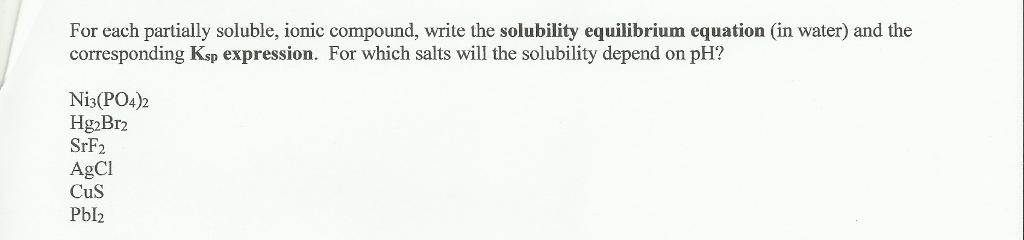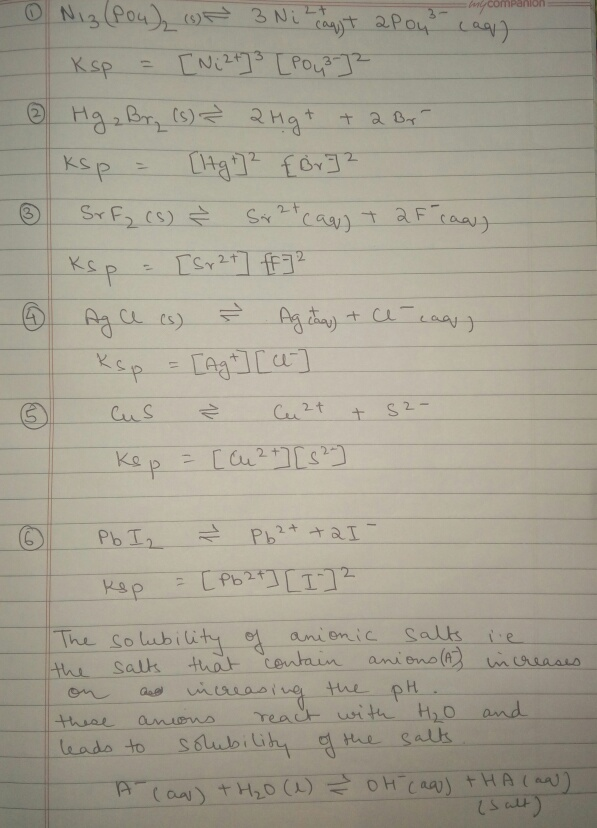# Question & Answer: For each partially soluble, ionic compound, write the solubility equilibrium equation (in water)…..For each partially soluble, ionic compound, write the solubility equilibrium equation (in water) and the corresponding K_sp expression. For which salts will the solubility depend on pH? Ni_3(PO_4)_2 Hg_2Br_2 SrF_2 AgCl CuS Pbl_2